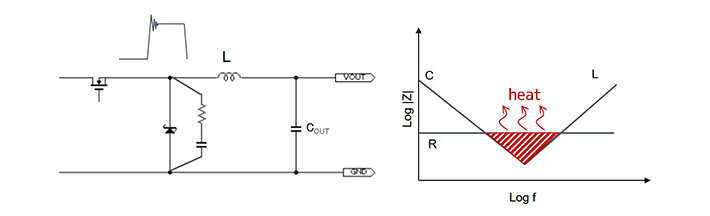Technical Information Site of Power Supply Design

2019.09.11 Switching Noise - EMC

# RC Snubber Circuit

Other Noise Countermeasures

As noise countermeasures for switching power supplies, we previously explained features and important points relating to methods using capacitors and inductors. In addition to these, however, there are a number of other methods for dealing with noise. Among these, we here explain RC snubber circuits, which are frequently used.

RC Snubber Circuit

An RC snubber circuit can be added with the objective of reducing spike voltage occurring at a switch node. In the following example, when a rectifying diode is turned off (when the high-side switch is on), the RC snubber circuit discharges the electric charge accumulated in the diode junction, the parasitic inductance, the parasitic capacitance, and the inductance in the PCB trace, converting the voltage into heat through a resistance to reduce spikes.As the RC values, generally R = 2Ω and C = 470 pF are used to start with, and actual measurements are performed to determine optimal values.

As one point to note, if the addition of a snubber circuit causes the switching transition to be more gradual, efficiency will be reduced. Hence it will be necessary to examine the trade-off between the noise level and efficiency.

Moreover, as it is assumed that the resistance will convert noise voltages into heat, attention must be paid to the allowable dissipation of the resistance. Resistance loss can be calculated using the following equation.

Loss＝C×VIN2×fSW

Snubber circuits are frequently used on the high side as well as on the low side. The Application Note "Snubber Circuit for Buck Converter IC（PDF）" provides details on snubber circuits, and should be referenced.

#### Key Points:

・RC snubber circuits reduce voltage spikes, occurring due to parasitic capacitances and parasitic inductances, by using resistors to convert the voltages into heat.

・The addition of a snubber circuit may possibly reduce circuit efficiency, and so the trade-off between noise level and efficiency must be studied.

・A resistance converts noise voltages into heat, and so attention must be paid to the allowable dissipation of the resistance.